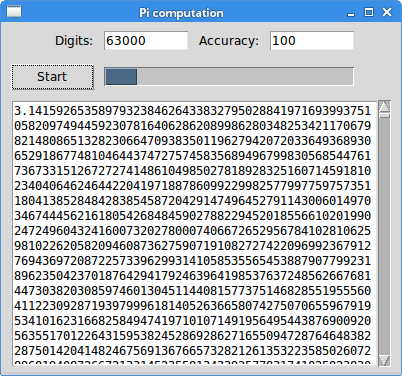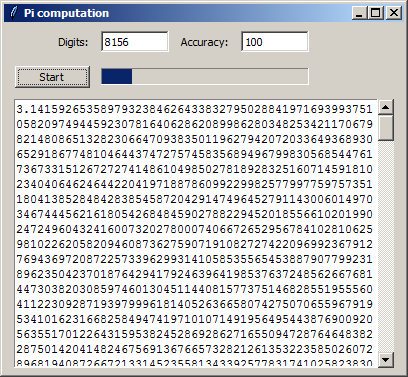# Long-running task in Tkinter

Tkinter uses a single-threaded event model. The main event loop and all the event handlers execute in one thread. Therefore, the event handlers must be very quick otherwise the user considers the application unresponsive. Long-running tasks must be executed outside the main event thread.

In our program we calculate the Pi number; the longer the number, the more time is needed for the calculation.

## Multiprocessing

We use the Python's `multiprocessing` module to execute a lengthy task in a separate process. This package supports spawning processes using an API similar to the `threading` module. The `multiprocessing` module works differently on Windows and Linux. On Linux, the `fork()` system call is leveraged while on Windows a `pickle` module is used. This has some important consequences. We split the code example into Linux and Windows programs.

There is one limitation of our program. It uses a `Queue` object for exchanging values between processes. This queue has a limit for the size of the value it can receive. The size is determined by the OS pipe. After exceeding this limit the program ends up in a deadlock. The largest Pi calculated on Windows 7 had 8156 digits. On Linux, we managed to calculate more than 60 000 digits of Pi.

## Calculating Pi on Linux

The following program calculates Pi on Linux.

calculate_pi.py
```#!/usr/bin/env python3
# -*- coding: utf-8 -*-

"""
ZetCode Tkinter e-book

This script produces a long-running task of calculating
a large Pi number, while keeping the GUI responsive.

Author: Jan Bodnar
Last modified: January 2016
Website: www.zetcode.com
"""

from tkinter import (Tk, BOTH, Text, E, W, S, N, END,
NORMAL, DISABLED, StringVar)
from tkinter.ttk import Frame, Label, Button, Progressbar, Entry
from tkinter import scrolledtext

from multiprocessing import Queue, Process
import queue
from decimal import Decimal, getcontext

DELAY1 = 80
DELAY2 = 20

class Example(Frame):

def __init__(self, parent, q):
Frame.__init__(self, parent)

self.queue = q
self.parent = parent
self.initUI()

def initUI(self):

self.parent.title("Pi computation")
self.pack(fill=BOTH, expand=True)

self.grid_columnconfigure(4, weight=1)
self.grid_rowconfigure(3, weight=1)

lbl1 = Label(self, text="Digits:")
lbl1.grid(row=0, column=0, sticky=E, padx=10, pady=10)

self.ent1 = Entry(self, width=10)
self.ent1.insert(END, "4000")
self.ent1.grid(row=0, column=1, sticky=W)

lbl2 = Label(self, text="Accuracy:")
lbl2.grid(row=0, column=2, sticky=E, padx=10, pady=10)

self.ent2 = Entry(self, width=10)
self.ent2.insert(END, "100")
self.ent2.grid(row=0, column=3, sticky=W)

self.startBtn = Button(self, text="Start",
command=self.onStart)
self.startBtn.grid(row=1, column=0, padx=10, pady=5, sticky=W)

self.pbar = Progressbar(self, mode='indeterminate')
self.pbar.grid(row=1, column=1, columnspan=3, sticky=W+E)

self.txt = scrolledtext.ScrolledText(self)
self.txt.grid(row=2, column=0, rowspan=4, padx=10, pady=5,
columnspan=5, sticky=E+W+S+N)

def onStart(self):

self.startBtn.config(state=DISABLED)
self.txt.delete("1.0", END)

self.digits = int(self.ent1.get())
self.accuracy = int(self.ent2.get())

self.p1 = Process(target=self.generatePi, args=(self.queue,))
self.p1.start()
self.pbar.start(DELAY2)
self.after(DELAY1, self.onGetValue)

def onGetValue(self):

if (self.p1.is_alive()):

self.after(DELAY1, self.onGetValue)
return
else:

try:
self.txt.insert('end', self.queue.get(0))
self.txt.insert('end', "\n")
self.pbar.stop()
self.startBtn.config(state=NORMAL)

except queue.Empty:
print("queue is empty")

def generatePi(self, queue):

getcontext().prec = self.digits

pi = Decimal(0)
k = 0
n = self.accuracy

while k < n:
pi += (Decimal(1)/(16**k))*((Decimal(4)/(8*k+1)) - \
(Decimal(2)/(8*k+4)) - (Decimal(1)/(8*k+5))- \
(Decimal(1)/(8*k+6)))
k += 1
print (self.p1.is_alive())

queue.put(pi)
print("end")

def main():

q = Queue()

root = Tk()
root.geometry("400x350+300+300")
app = Example(root, q)
root.mainloop()

if __name__ == '__main__':
main()
```

The program calculates the Pi number. The calculation takes a long time to finish. To remain responsive, the calculation is placed in a separate process.

```self.pbar = Progressbar(self, mode='indeterminate')
self.pbar.grid(row=1, column=1, columnspan=3, sticky=W+E)
```

We have a `Progressbar` widget, which is in progress during the calculation. This way we tell the user that the application is still running.

```def onStart(self):

self.startBtn.config(state=DISABLED)
self.txt.delete("1.0", END)
...
```

At the start of the calculation, we disable the Start button and delete the contents of the text widget.

```self.digits = int(self.ent1.get())
self.accuracy = int(self.ent2.get())
```

There are two important numbers used in the calculation of the Pi number. The `digits` value determines the lenght of the number. The `accuracy` number controls the precision of the given formula.

```self.p1 = Process(target=self.generatePi, args=(self.queue,))
self.p1.start()
```

A new `Process` is started. The target is the `generatePi()` method, which computes the lengthy task. The other argument is the queue, which is an object used to communicate between processes.

```self.after(DELAY1, self.onGetValue)
```

We start a timer. The timer periodically checks if the task is already finished.

```def onGetValue(self):

if (self.p1.is_alive()):

self.after(DELAY1, self.onGetValue)
return
```

With the `is_alive()` method, we check is the task is still running.

```else:

try:
self.txt.insert('end', self.queue.get(0))
self.txt.insert('end', "\n")
self.pbar.stop()
self.startBtn.config(state=NORMAL)

except queue.Empty:
print("queue is empty")
```

When the task has ended, we get the computed number from the queue, insert it into the text widget, and stop the progress bar. Also, the button is enabled.

```def generatePi(self, queue):

getcontext().prec = self.digits

pi = Decimal(0)
k = 0
n = self.accuracy

while k < n:
pi += (Decimal(1)/(16**k))*((Decimal(4)/(8*k+1)) - \
(Decimal(2)/(8*k+4)) - (Decimal(1)/(8*k+5))- \
(Decimal(1)/(8*k+6)))
k += 1

queue.put(pi)
print("end")
```

The `generatePi()` method contains the formula to calculate the Pi number. There are several formulas available; we have used the Bailey–Borwein–Plouffe formula. At the end of the calculation, the number is put into the queue. It is importat that we do not touch anything in the main GUI thread from this method. Note the use of the `Decimal`; the Python's float data type is not precise enough for our calculations.Figure: Calculating Pi on Linux

The image shows a calculated Pi having 63000 digits.

## Calculating Pi on Windows

The following program calculates Pi on Windows. Since on Windows `multiprocessing` uses `pickle` module, it inherits its limitations. The `generatePi()` must be a top-level module function and the queue must be a global object.

calculate_pi_windows.py
```#!/usr/bin/env python3
# -*- coding: utf-8 -*-

"""
ZetCode Tkinter e-book

This script produces a long-running task of calculating
a large Pi number, while keeping the GUI responsive.
This is an example written for Windows.

Author: Jan Bodnar
Last modified: January 2016
Website: www.zetcode.com
"""

from tkinter import (Tk, BOTH, Text, E, W, S, N, END,
NORMAL, DISABLED, StringVar)
from tkinter.ttk import Frame, Label, Button, Progressbar, Entry
from tkinter import scrolledtext

from multiprocessing import Process, Manager, Queue
from queue import Empty
from decimal import Decimal, getcontext

DELAY1 = 80
DELAY2 = 20

# Queue must be global
q = Queue()

class Example(Frame):

def __init__(self, parent):
Frame.__init__(self, parent, name="frame")

self.parent = parent
self.initUI()

def initUI(self):

self.parent.title("Pi computation")
self.pack(fill=BOTH, expand=True)

self.grid_columnconfigure(4, weight=1)
self.grid_rowconfigure(3, weight=1)

lbl1 = Label(self, text="Digits:")
lbl1.grid(row=0, column=0, sticky=E, padx=10, pady=10)

self.ent1 = Entry(self, width=10)
self.ent1.insert(END, "4000")
self.ent1.grid(row=0, column=1, sticky=W)

lbl2 = Label(self, text="Accuracy:")
lbl2.grid(row=0, column=2, sticky=E, padx=10, pady=10)

self.ent2 = Entry(self, width=10)
self.ent2.insert(END, "100")
self.ent2.grid(row=0, column=3, sticky=W)

self.startBtn = Button(self, text="Start",
command=self.onStart)
self.startBtn.grid(row=1, column=0, padx=10, pady=5, sticky=W)

self.pbar = Progressbar(self, mode='indeterminate')
self.pbar.grid(row=1, column=1, columnspan=3, sticky=W+E)

self.txt = scrolledtext.ScrolledText(self)
self.txt.grid(row=2, column=0, rowspan=4, padx=10, pady=5,
columnspan=5, sticky=E+W+S+N)

def onStart(self):

self.startBtn.config(state=DISABLED)
self.txt.delete("1.0", END)

digits = int(self.ent1.get())
accuracy = int(self.ent2.get())

self.p1 = Process(target=generatePi, args=(q, digits, accuracy))
self.p1.start()
self.pbar.start(DELAY2)
self.after(DELAY1, self.onGetValue)

def onGetValue(self):

if (self.p1.is_alive()):

self.after(DELAY1, self.onGetValue)
return
else:

try:

self.txt.insert('end', q.get(0))
self.txt.insert('end', "\n")
self.pbar.stop()
self.startBtn.config(state=NORMAL)

except Empty:
print("queue is empty")

# Generate function must be a top-level module funtion
def generatePi(q, digs, acc):

getcontext().prec = digs

pi = Decimal(0)
k = 0
n = acc

while k < n:
pi += (Decimal(1)/(16**k))*((Decimal(4)/(8*k+1)) - \
(Decimal(2)/(8*k+4)) - (Decimal(1)/(8*k+5))- \
(Decimal(1)/(8*k+6)))
k += 1

q.put(pi)

def main():

root = Tk()
root.geometry("400x350+300+300")
app = Example(root)
root.mainloop()

if __name__ == '__main__':
main()
```

This code example was rewritten for Windows.Figure: Calculating Pi on Windows

The image shows a calculated Pi having 8156 digits. Computing larger numbers ends up in a deadlock because of the limitations of the Queue object and the OS pipe on Windows.

In this article, we have shown how to create long-running tasks in Tkinter and Python 3; we have utilized the `multiprocessing` module. During the development of the program we have encountered some technical limitations. For more information about Tkinter, you can read the Tkinter tutorial.

ZetCode last modified January 26, 2016 © 2007 - 2019 Jan Bodnar Follow on Facebook